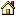PHPBuddy.comPHP Function Lookup:

 Categories

 Top Rated Articles

 Site RelatedArticle Added on: April 15, 2002Overview: We will write first PHP code, this section also covers basic PHP syntax, including variable usage.

Before you can work with PHP you need to install PHP on your computer if you havenít installed PHP yet, now is a good time to install PHP.

First we will make a simple HTML page

``` <html> <body> Welcome to PHP tutorial </body> </html>```

Okay the above HTML page doesn't do anything special but just outputs Welcome to PHP tutorial on the browser, now lets add some PHP tags to it.

Note: All PHP code is written between <?php and ?> tags, now lets write "Welcome to PHP tutorial" using PHP.

``````<html>
<body>

<?php
echo "Welcome to PHP tutorial";
?>

</body>
</html>``````
Okay the above PHP script will output Welcome to PHP tutorial note that we use the echo construct of PHP, the echo function simply outputs the content to the browser.

So if we wanted to write "Welcome" we would write echo "Welcome";

Note Have you noticed the ; at the end of the statment, Each PHP instruction must end with a semicolon.

Introduction to Variables
Let me introduce you to PHP variables, variables help you store and retrive data.

• The name of the variable can consist of letters and numbers, but must begin with a letter. It can also contain special characters like underscore '_', see some examples below
``` \$site = 'phpbuddy.com' // valid variable \$4site = 'not yet'; // invalid variable starts with a number \$_4site = 'not yet'; // valid variable starts with an underscore ```
Some more examples
``` \$testvariable = 1 + 1; // Assigns a value of 2. \$testvariable = 1 ń 1; // Assigns a value of 0. \$testvariable = 2 * 2; // Assigns a value of 4. \$testvariable = 2 / 2; // Assigns a value of 1. ```
Okay to make things easier I will show you an example here we will create a variables \$a, \$b and multiply them and store the value in \$c, just see it's so simple
``````
<?php
\$a = 5;  //we created a variable a and assigned it a value of 5
\$b = 2;  //we created a variable b and assigned it a value of 2

\$c = \$a * \$b;  //we multiply varaible a and b and store the value in c
echo \$c;  //we print the content of variable c which is 10
?>
``````

PHP variables can hold strings, dynamic data, PHP variables are case sensitive \$a is not the same as \$A

``````
<?php
\$a = "Welcome to PHP ";  //\$a holds the string Welcome to PHP
\$A = 4;  //\$A holds the value 4

echo "Variable a conatains: \$a";
echo "Variable A contains: \$A";

echo "\$a \$A";  //outputs both variables \$a and \$A
?>
``````

Okay now we know what are variable and how to work with them, In the next part we learn how to add logic to our programms.